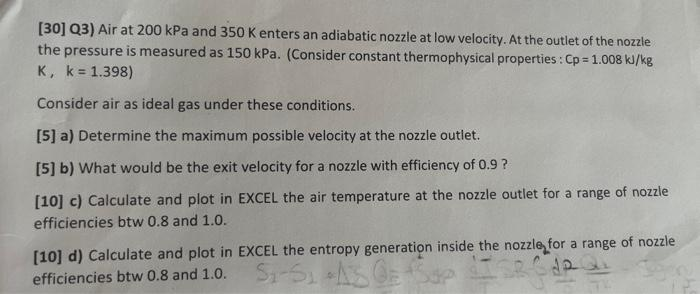# (Solved):  Q3) Air at 200kPa and 350K enters an adiabatic nozzle at low velocity. At the outlet of the ...Q3) Air at and enters an adiabatic nozzle at low velocity. At the outlet of the nozzle the pressure is measured as . (Consider constant thermophysical properties: Consider air as ideal gas under these conditions.  a) Determine the maximum possible velocity at the nozzle outlet.  b) What would be the exit velocity for a nozzle with efficiency of ?  c) Calculate and plot in EXCEL the air temperature at the nozzle outlet for a range of nozzle efficiencies btw and .  d) Calculate and plot in EXCEL the entropy generation inside the nozzle, for a range of nozzle efficiencies btw and .

We have an Answer from Expert